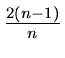Next: 4.6.3 Credit Balance Up: 4.6 Amortized Algorithm Analysis Previous: 4.6.1 Example of Sorting

## 4.6.2 Example of Tree Traversal (Inorder)

• Let the cost of visiting a vertex be the number of branches traversed to reach that vertex from the last one visited.

Best case cost = 1 Worst case cost = n -1

• If we amortize the cost over a traversal of the entire binary tree, then the cost of going from each vertex to the next is less than 2. To see why, note that every binary tree with n vertices has exactly n - 1 branches and a complete traversal of the tree goes over each branch exactly twice. Thus the total number of steps in a full traversal = 2(n - 1).

• Amortized number of steps from one vertex to the next =< 2

eEL,CSA_Dept,IISc,Bangalore